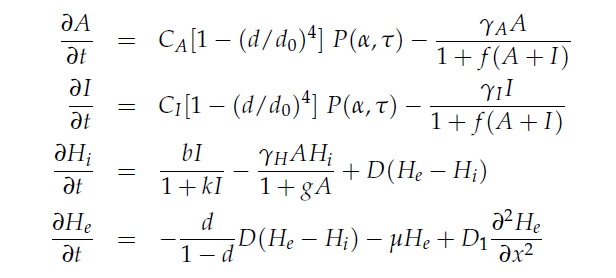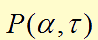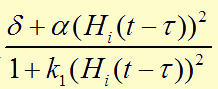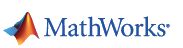# Team:XMU-China/Content modeling

### Modeling

#### Mathematical model

We modeled the oscillate gene circuit (without ndh gene) based on the paper "A synchronized quorum of genetic clocks". In that, the author characterized the oscillator by using the four delay differential equations (DDEs), which are the basic of our modeling. DDE can perfectly simulate our circuit due to its powerful function about solving the dynamic change in biological processes.In the first two equations,item is a Hill function equaling to, which describes the delayed reactions about the complex processes in the cells (transcription, translation, maturation, etc.). This Hill function takes the history of the system into account, i.e. the concentration of AHL at the time it binds to LuxR to form the activation complex.
This model does not include an equation for LuxR assuming that it is constitutively produced at a constant level. All parameters are normalized for the convenience of analysis.

#### Modeling in MATLAB

We used MATLAB to help us solve the DDEs and draw the graphs. In addition, we developed a GUI user interface after finishing the main DDE code. The user interface makes people easier to modify and adjust the parameters without recoding the .m files and any MATLAB language knowledge. We called this software "Gene OS" which is short for Gene oscillate simulator.

See more in Software